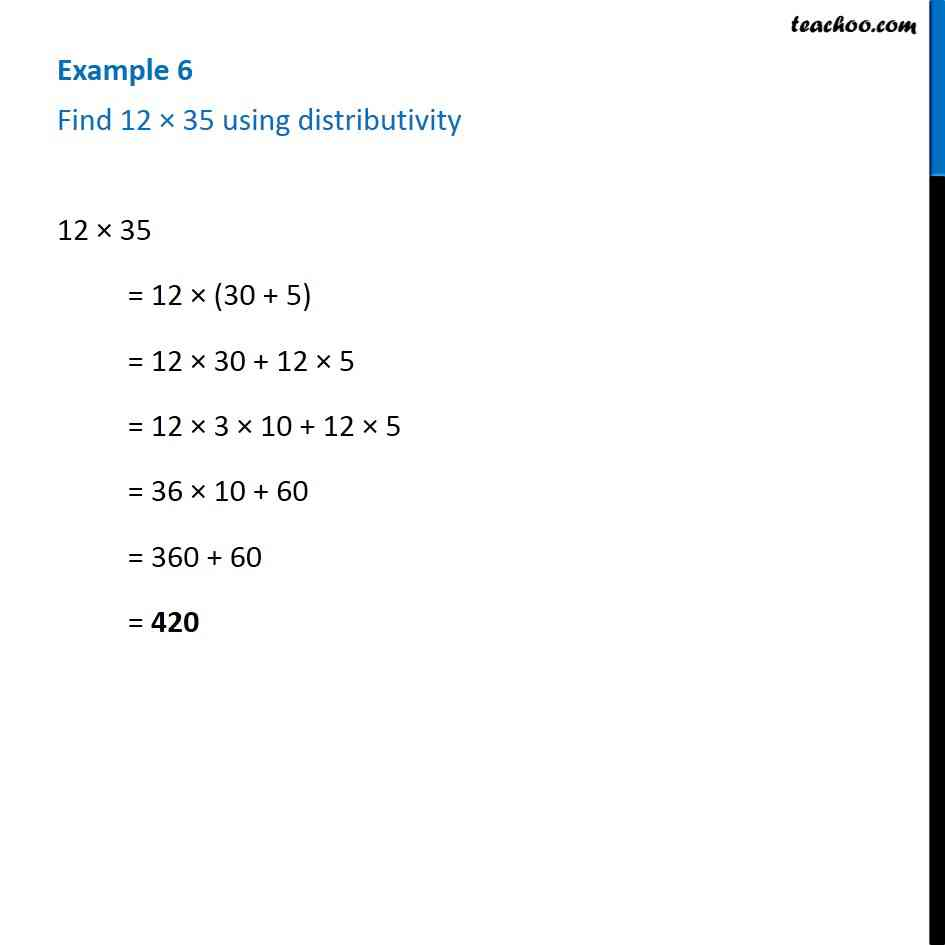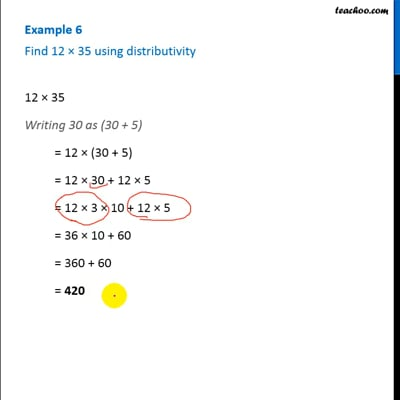Examples

Chapter 2 Class 6 Whole Numbers
Serial order wiseThis video is only available for Teachoo black users

### Transcript

Example 6 - Chapter 2 Class 6 Whole Numbers - NCERT Book Find 12 × 35 using distributivity 12 × 35 = 12 × (30 + 5) = 12 × 30 + 12 × 5 = 12 × 3 × 10 + 12 × 5 = 36 × 10 + 60 = 360 + 60 = 420